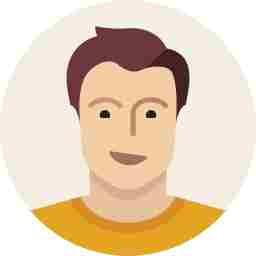## In CLAT, to score good marks in Quantitative Aptitude, one should have good Command over elementary Mensuration. In Mensuration, you should have some basic knowledge of Area & Volume of Rectangle, Square, Parallelogram, rhombus, Cube, Cuboid, Cylinder, Sphere, Cone. How to Solve questions related to Mensuration: Tricks and Practice Questions Formulas related to Mensuration A. Cuboid    … Read More »

In CLAT, to score good marks in Quantitative Aptitude, one should have good Command over elementary Mensuration. In Mensuration, you should have some basic knowledge of Area & Volume of Rectangle, Square, Parallelogram, rhombus, Cube, Cuboid, Cylinder, Sphere, Cone. How to Solve questions related to Mensuration: Tricks and Practice Questions Formulas related to Mensuration A. Cuboid l: length b:breadth h:height Lateral surface area (LSA)...

In CLAT, to score good marks in Quantitative Aptitude, one should have good Command over elementary Mensuration. In Mensuration, you should have some basic knowledge of Area & Volume of Rectangle, Square, Parallelogram, rhombus, Cube, Cuboid, Cylinder, Sphere, Cone.

## How to Solve questions related to Mensuration: Tricks and Practice Questions

### A. CuboidLateral surface area (LSA) = 2h(l+b)

Total surface area (TSA)= 2 (lb +bh+hl

Volume=LxBxH

### B. Cubea: Edge of the cube

Lateral surface area (LSA) =4a2

Total surface area (TSA)=6a2

Volume=a3

### C. CylinderLateral surface area (LSA) = 2πrh

Total surface area (TSA)= 2πrh+2πr2

Volume=πr2h

### D. Conel: slant height r: radius h: height

Lateral surface area (LSA) = πrl

Total surface area (TSA)= πrl+πr2

Volume=1/3πr2h

### E. SphereLateral surface area (LSA) = 4πr2

Total surface area (TSA)= 4πr2

Volume=4/3πr3

### F. HemisphereLateral surface area (LSA) = 2πr2

Total surface area (TSA)= 3πr2

Volume=2/3π3

## Tips to solve Mensuration based questions

1. Try to learn square and cube of 1 to 30, it will help you to avoid extra calculations and save time.
2. Try to learn all formulas of mensuration on tips, as by using formulas you can avoid calculations.
3. In question, if the values are given in decimal then use pie value as 3.14, if the values are in fraction then use pie value as 22/7.
4. In mensuration it is not essential to find all the values to get to an answer, always check that which area is mentioned to find. For example, if in a question there is a cone made in the square and a question is asked to you regarding the volume of the cone, then you should find only the volume of the cone not the volume of the square.
5. To solve questions quickly, as most of the students just read the questions and start to solve them, but they should also analyze the diagram of the questions properly.
6. In mensuration to find the volume of a combination of two or more basic solids, we should add their volumes.
7. In mensuration to find the surface areas of a combination of two or more solids, it is not necessary to add their surfaces directly.

## Sample question

A room having the dimensions as mentioned in the chart below has a table, a bed, and a chair. There are three books kept on the table (side by side) and also a lamp having a radius, 3.5 inches. Now, based on the information given below answer the following?

 S.No Object Length Breadth Height 1 Room 18 12 12 2 Bed 3.5 6 3 3 Table 3 4 4 4 Chair 2 2.5 4 5 Books 12 6 3

### 1. What would be the area of the room that is not covered by any object as mentioned above?

1. 176.5 sq. ft
2. 178 sq. ft
3. 367 sq. ft
4. 245.5 sq. ft.

### 2. What is the area of the table which is uncovered by any of the objects?

1. 1656 sq. inch
2. 52 sq. inch
3. 52 sq. inch
4. 1512 sq. inch.

1. 18
2. 11
3. 21

1. 2,00,736
2. 20,736
3. 11,736
4. 17,522.

### 5. How many chairs can be put inside the room to cover the entire floor area of the room?

1. 40
2. 21
3. 43
4. 18.